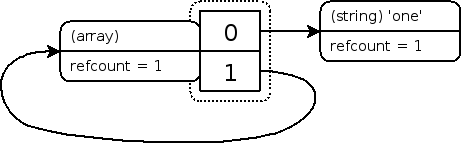PHP 8.1.26 Released!

## 引用计数基础

PHP 变量存储在称为“zval”的容器中。zval 容器除了变量的类型和值之外，还包含两个额外的信息位。第一个是“is_ref”，是布尔值，表示变量是否是“引用集合”的一部分。通过这个位，PHP 引擎知道如何区分普通变量和引用。由于 PHP 允许用户自定义引用，通过 & 运算符创建引用，zval 容器还有内部引用计数机制来优化内存使用。第二个是“refcount”，表示有多少个变量名（也称为符号）指向这个 zval 容器。所有符号都存储在一个符号表中，每个作用域都有一个符号表。主脚本（即通过浏览器请求的脚本）有一个作用域，每个函数或方法也有一个作用域。

`<?php\$a = "new string";?>`

`<?php\$a = "new string";xdebug_debug_zval('a');?>`

```a: (refcount=1, is_ref=0)='new string'
```

`<?php\$a = "new string";\$b = \$a;xdebug_debug_zval( 'a' );?>`

```a: (refcount=2, is_ref=0)='new string'
```

`<?php\$a = "new string";\$c = \$b = \$a;xdebug_debug_zval( 'a' );\$b = 42;xdebug_debug_zval( 'a' );unset( \$c );xdebug_debug_zval( 'a' );?>`

```a: (refcount=3, is_ref=0)='new string'
a: (refcount=2, is_ref=0)='new string'
a: (refcount=1, is_ref=0)='new string'
```

### 复合类型

`<?php\$a = array( 'meaning' => 'life', 'number' => 42 );xdebug_debug_zval( 'a' );?>`

```a: (refcount=1, is_ref=0)=array (
'meaning' => (refcount=1, is_ref=0)='life',
'number' => (refcount=1, is_ref=0)=42
)
````<?php\$a = array( 'meaning' => 'life', 'number' => 42 );\$a['life'] = \$a['meaning'];xdebug_debug_zval( 'a' );?>`

```a: (refcount=1, is_ref=0)=array (
'meaning' => (refcount=2, is_ref=0)='life',
'number' => (refcount=1, is_ref=0)=42,
'life' => (refcount=2, is_ref=0)='life'
)
````<?php\$a = array( 'meaning' => 'life', 'number' => 42 );\$a['life'] = \$a['meaning'];unset( \$a['meaning'], \$a['number'] );xdebug_debug_zval( 'a' );?>`

```a: (refcount=1, is_ref=0)=array (
'life' => (refcount=1, is_ref=0)='life'
)
```

`<?php\$a = array( 'one' );\$a[] =& \$a;xdebug_debug_zval( 'a' );?>`

```a: (refcount=2, is_ref=1)=array (
0 => (refcount=1, is_ref=0)='one',
1 => (refcount=2, is_ref=1)=...
)
``````(refcount=1, is_ref=1)=array (
0 => (refcount=1, is_ref=0)='one',
1 => (refcount=1, is_ref=1)=...
)
```### User Contributed Notes 6 notes

17
Anonymous
8 years ago
`If a variable is not present in the current scope xdebug_debug_zval　will return null.`
13
Anonymous
8 years ago
`There seems to be no way to inspect the reference count of a specific class variable but you can view the reference count of all variables in the current class instance with xdebug_debug_zval('this');`
skymei at skymei dot cn
3 years ago
`\$a = 'new string';\$b = 1;xdebug_debug_zval('a');xdebug_debug_zval('b');ouputs with PHP 7.3.12 (cli) a: (interned, is_ref=0)='new string'b: (refcount=0, is_ref=0)=1`
shkarbatov at gmail dot com
5 years ago
`Result of "Example #8 Adding the array itself as an element of it self" will be another for PHP7:a: (refcount=2, is_ref=1)=array ( 0 => (refcount=2, is_ref=0)='one', 1 => (refcount=2, is_ref=1)=...)insted of:a: (refcount=2, is_ref=1)=array ( 0 => (refcount=1, is_ref=0)='one', 1 => (refcount=2, is_ref=1)=...)Internal value representation in PHP 7:https://nikic.github.io/2015/05/05/Internal-value-representation-in-PHP-7-part-1.html`
yuri1308960477 at gmail dot com
4 years ago
`my php versoin : HP 7.1.25 (cli) (built: Dec 7 2018 08:20:45) ( NTS )\$a = 'new string';\$b = 1;xdebug_debug_zval('a');xdebug_debug_zval('b');output:a: (refcount=2, is_ref=0)='new string'b: (refcount=0, is_ref=0)=1if \$a is a string value, 'refcount' equal 2 by defalut.`
chxt2011 at 163 dot com
4 years ago
`my php version is PHP 7.1.6 (cli), when I run \$a = 'new string';\$b = 1;xdebug_debug_zval('a');xdebug_debug_zval('b');it shows:a: (refcount=0, is_ref=0)='new string'b: (refcount=0, is_ref=0)=1`